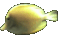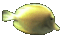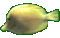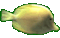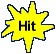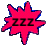Derivative Product Rule with Sine Cosine & Power Rules    Math Help Game Tips:

- The derivative Product Rule is used on the product of two relations such as the product y=uv.
In general, the Product Rule states that the derivative of the product function y=uv  is    y'=uv'+u'v.
- For example, the unsimplified derivative of y=(x3x5)  is    y'=(x3)(5x4)+(3x2)(x5).
Note that the symbols  dy/dx,  y' and  f '(x)  all represent the same derivative of y=f(x).

- This game uses the Product Rule   dy/dx = (u)(dv/dx) + (du/dx)(v)
and also requires 4 other basic derivative rules:
the Power Rule, the Sine Rule, the Cosine Rule, and the Constant times a Function Rule.

1) The derivative Power Rule for y=xn yields the derivative  dy/dx = nxn-1.
For example, the derivatives of y=x4 and s(t)=t6 are respectively  dy/dx=4x3 and s'(t)=6t5.

2) The derivative Sine Rule for y=sin(x) yields the derivative  dy/dx = cos(x).
This means that the derivative of y=sin(x) is y'=cos(x).

3) The derivative Cosine Rule for y=cos(x) yields the derivative  dy/dx =-sin(x).
This means that the derivative of y=cos(x) is y'=-sin(x).

4) The derivative rule for a Constant times a Function such as y=cu yields the derivative dy/dx = c(du/dx).
For example, the derivatives of y=8x4 and g(t)=5sin(t) are respectively y'=32x3 and g'(t)=5cos(t).

- Three more examples of the Product Rule y'=uv'+u'v follow:
The derivative of y=(x3sin(x))  is    y'=(x3)(cos(x))+(3x2)(sin(x)).
The unsimplified derivative of y=2x3[8cos(x)]  is    y'=2x3[-8sin(x)]+6x2[8cos(x)].
The derivative of y=(2sin(x))(8cos(x))  is    y'= -16sin(x)sin(x)+16cos(x)cos(x).
which simplifies to y'= -16sin2(x)+16cos2(x)  or   y'= 16[cos2(x)-sin2(x)].

- Your Game Score is reduced by the number of fish hits.
- To slow the game speed repeat tap/click on the word Slider.
- To increase the game speed repeat tap/click on the word Math.
- Speed can also be adjusted with a keyboard's - and + keys.

- Refresh/Reload the web page to restart the game.